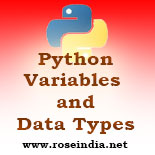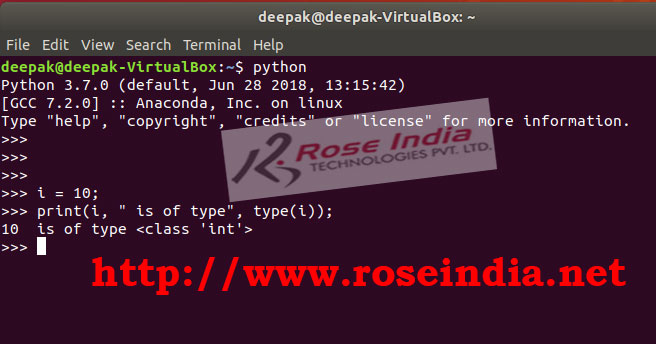# Python variables and data types

In this section we will learn about variables and data types in Python Programming Language.## Python Tutorials: Learning variables and data types in Python with examples

The variables and data types are essential topics in Python programming language. Programmers should learn to use various data types in Python Programming Language. You should have solid understanding of using various Python data types and its use in creating right variables to meet our business application requirements. In this section we will learn variables and data types of Python Programming language.

In this we will be discussing variable and data types in Python in detail. You will find examples of declaring variable of all these data types. This tutorial is for beginner in Python and it gives you detailed introduction to variables and data types in Python with example programs.

### Python Data Types

When you create a variable to store some value in your program (in memory during runtime) then program execution library creates an object in memory and associates a type to the data. So, every data in Python is associated with a type called data type.

So, every data in Python is associated with a type called data type and it is determined automatically when data is assigned to it first time. Once the data is assigned and � data type is associated it is available for use in the program.  You can't assign a different kind of data to a variable. For example if variable is of int type then you can't assign a string data to it.Everything in Python is an object and data types are instance of classes. Variables are instance (object) of any of these classes. Here is an example of int variable in Python:

``````
i = 10;
print(i, " is of type", type(i));
``````

If you run the program you will get following output:

``````
>>> i = 10;
>>> print(i, " is of type", type(i));
10  is of type <class 'int'>
>>>
``````

Here is screen shot of program execution in Python shell:In the above program we have declared integer type variable in Python by assigning int value to it. Then in our program we have printed the value and type of variable. The type() function is used in Python to print the type of a variable.

### Data Types in Python Programming Language

Now we will see the data types which come with the Python Programming language. Here is the list of data types in Python:

#### 1. Python Numbers

The Python numbers include int, float and complex classes.

#### 2. Python List

In Python list represents an ordered sequence of items.

#### 3. Python Tuple

The Python Tuple is immutable ordered sequence of items.

#### 4. Python Strings

The Strings in Python is used to store sequence of Unicode characters.

#### 5. Python Set

Set is used to store Unicode items and it is unordered collection.

#### 6. Python Dictionary

Python Dictionary is used to store key-value pair data in a Python program. Dictionary is also an unordered collection of items which stores value in key-value pair.

### Python Variables

Variables are the object of any class or data type which hold the data in while program is executing. This is the memory location to hold the data in program and it has a type associated with it.

Python programming language is dynamically typed and there is no requirement of assigning the data type to a variable during declaration. When you assign a value to it, the programming language detects the type of data and determines the data type for the variable

Python variable name convention follows the naming convention of identifiers. You can check more at https://www.roseindia.net/python/python-keywords-and-identifiers.shtml.

In a nutshell you can use any characters (a to z and A to Z) and numbers (0-9) in any combination for naming a variable. It may contain numbers, characters and underscore (_). But the variable name should not start with a number. Variable name can start with underscore (_).

In the following code you will find the example of declaring all types of variable in Python:

``````#Integer declaration
i = 108

print(i, " is of type", type(i));

# Declaring long integer
j = 108**100

print(j, " is of type", type(j));

# Declaring double float in Python
k = 10.82
print(k, " is of type", type(k));

# Declaring string in Python
l = "Welcome to Python"

print(l, " is of type", type(l));

# Declaring list in python
m = [1,0,8]

print(m, " is of type", type(m));

# Declaring tuple in Python
n = (1,0,8)

print(n, " is of type", type(n));

# Declaring Dictionary  in Python
book_dict = {"name":"Python Book", "price":"100"}
print(book_dict, " is of type", type(book_dict));``````

Here is the output of program:

``````[email protected]\$ python
Python 3.7.0 (default, Jun 28 2018, 13:15:42)
[GCC 7.2.0] :: Anaconda, Inc. on linux
>>> #Integer declaration
... i = 108
>>>
>>> print(i, " is of type", type(i));
108  is of type <class 'int'>
>>>
>>> # Declaring long integer
... j = 108**100
>>>
>>> print(j, " is of type", type(j));
219976125634128528197368544431729364618631012313582745611466368
33168583150866902757543292705894263836529214935144820454164771
533186395907429212541162286744128795806157605188413764
2597711028147158438117376  is of type <class 'int'>
>>>
>>> # Declaring double float in Python
... k = 10.82
>>> print(k, " is of type", type(k));
10.82  is of type <class 'float'>
>>>
>>> # Declaring string in Python
... l = "Welcome to Python"
>>>
>>> print(l, " is of type", type(l));
Welcome to Python  is of type <class 'str'>
>>>
>>> # Declaring list in python
... m = [1,0,8]
>>>
>>> print(m, " is of type", type(m));
[1, 0, 8]  is of type <class 'list'>
>>>
>>> # Declaring tuple in Python
... n = (1,0,8)
>>>
>>> print(n, " is of type", type(n));
(1, 0, 8)  is of type <class 'tuple'>
>>>
>>> # Declaring Dictionary  in Python
... book_dict = {"name":"Python Book", "price":"100"}
>>> print(book_dict, " is of type", type(book_dict));
{'name': 'Python Book', 'price': '100'}  is of type <class 'dict'>
>>>
>>>
``````

In the above example you learned to define various types of variable in Python.